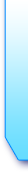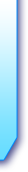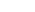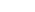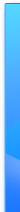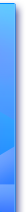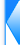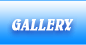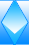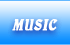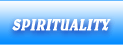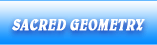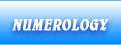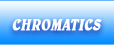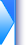### Numerology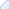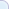Golden number -...Zero - the number of...One - the unique God...Two - the number of...Three - the number of...Four - number of...Five - the number of...Six - the number of...Seven - the number...Eight - the number...Nine - the number of...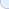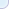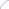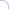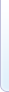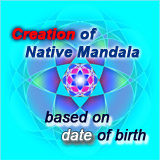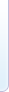# Golden number - universal Divine proportion

At the beginning of creation The Unique God divided Himself into two parts in a proportion very well defined in such a way the One Divine to rediscover and to reflect Himself on each side.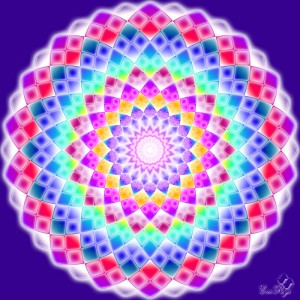Only in this way can be perfectly symmetrical creation and harmony in itself. This principle of division was repeated indefinitely, on several levels in the generation of Creation.

Mathematically we can find a number that corresponds to the principle of primary division equalizing reports obtained from the first division. More specifically if you divide a segment into two parts, the first part a denote with a and on the other with b must have 1/a=a/b, because b=1-a, get on the formula 1/a=a/1-a, otherwise writing 1/a-1=a, from this formula we want to find the ratio x=1/a, meaning x-1=1/x brings us to the equation x*x-x-1=0. The solution is 1,618… number that represents the golden number which can be found in the golden triangle of Pythagoras, the pentagon, the golden ellipse or spiral gold in the Fibonacci sequence which represents the natural growth.

This special ratio is found almost everywhere in nature, in the human body and art. This is the principle that underlies the generation of fractals. Explain the design principle of everything in part and part in everything. It is primordial and universal law of harmony.

It helps us to put in balance and harmony organs and body functions. Therefore each organ will work in harmony with the whole body.

Date: 2013-04-05

Keywords: represents, divine, proportion, golden, principle, pentagon, formula, himself, creation, number, universal, parts, ratio, division